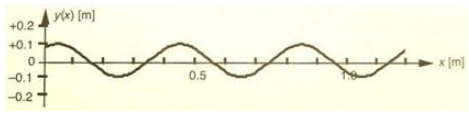# Problem: A machine tugs sideways on an elastic rope with a frequency of 4.5 Hz. A graph of the transverse displacements of this rope wave at t=1.0s as a function of position is shown below.a. What is the velocity of waves on this rope?b. If the frequency of the machine tugging on the rope is doubled to f=9.0 Hz yet the tension in the rope remains the same, what is the new wavelength of waves on this rope? What is the new velocity of waves on this rope?

###### FREE Expert Solution

Velocity:

$\overline{){\mathbf{v}}{\mathbf{=}}{\mathbf{f}}{\mathbf{\lambda }}}$

(a)

The distance from crest to crest if 0.4 m

λ = 0.4 m

f  = 4.5 Hz

90% (397 ratings)###### Problem Details

A machine tugs sideways on an elastic rope with a frequency of 4.5 Hz. A graph of the transverse displacements of this rope wave at t=1.0s as a function of position is shown below.a. What is the velocity of waves on this rope?

b. If the frequency of the machine tugging on the rope is doubled to f=9.0 Hz yet the tension in the rope remains the same, what is the new wavelength of waves on this rope? What is the new velocity of waves on this rope?